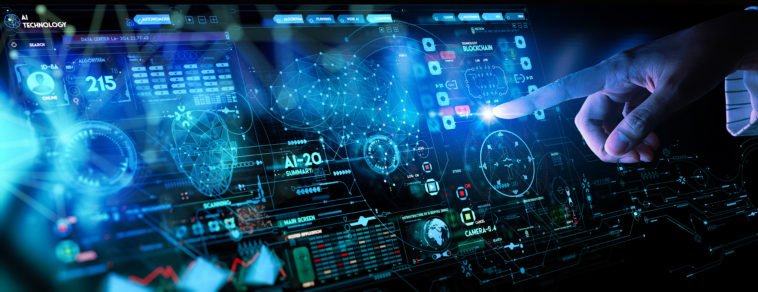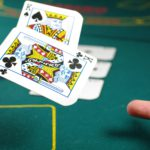# How To Measure Error In Machine Learning ModelsThere are several things a data expert must do when building a machine learning (ML) model. Among these things, measuring error is perhaps one of the essential parts of the process, and for good reasons. First, it allows you to judge the quality of your model. Second, you can compare your model with other models that use different parameters, allowing you to pick one that performs better.

On that note, it’s crucial to have a set procedure to measure the error in your ML models, but that’s easier said than done. For starters, there are two types of errors: human errors and errors made by the model. It’s common to mistake one for the other. Moreover, there are different types of machine learning models, each of which has varying metrics.

## 3 Common Types Of Machine Learning Model

Before anything else, it’s essential to understand that the process of measuring error would vary according to the model’s type. For your reference, here are three common types of ML models:

• Regression

This is perhaps the most commonly-used concept for ML models. It involves predicting the output value using an already known value, which is also known as the input.

For example, you can use regression to predict the price of gold in 2025 by using its current price. Of course, it’s impossible to accurately predict the future, so an error is inevitable.

• Binary Classification

As the name suggests, binary classification models aim to predict the classification of the subject of interest. For instance, if you’re tired of spam emails, you can create a binary classification model to determine if an email is a spam or a legitimate message. Since it’s binary, it only involves two classifications.

• Multiclass Classification

Multiclass classification is similar to the previous type, except there aren’t two classifications, but several. Using the earlier example, you can use multiclass classification to predict if an email belongs to Blacklisted, Spam, or Important.

Apart from the fact that error analysis goes different for each type, knowing the classification of your model can also help you determine the best way to minimize the error.

Custom loss functions, for example, have different implementations according to whether your model deals with regression or classification, like binary or categorical cross-entropy. If you’re curious about the custom loss function, Cnvrg’s article on it would be an excellent place to start.

For now, take a look at how to measure error for each type of ML model.

### Regression Models

Regression models are where you’ll most likely encounter issues when measuring error. This is mainly because it’s not as straightforward as the other two types.

The good news is that data experts have come up with several ways to measure the error in a regression machine learning model. One particular method is looking at the Root Mean Squared Error (RMSE), although others often only look at the Mean Squared Error (MSE).

The RMSE is a metric that determines how far the predictions were from the actual values, hence a good metric for measuring error. You can calculate the RMSE by following these steps:

1. Subtract the predicted values and their actual values, so you can get the difference.
2. Compute for the square of each difference.
3. Calculate the average of the values you get from step 2.
4. Take the square root of what you get from step 3.

The RMSE is the square root of MSE, so you can stop at step 3 to get the MSE. But since you need to measure error, it’s best to proceed to the last step. If you want your model to be more accurate, you want to get an RMSE value closer to zero. The closer it is, the better.

### Binary Classification Models

You might’ve already guessed, but measuring error in binary classification models is relatively more manageable than the previous type. You simply compare the number of times it successfully predicted and the number of times it failed. Naturally, the higher the success rate, the more accurate your model is, and vice versa.

There are several other ways to measure error, but this is generally the easiest and simplest way to determine how error-prone your model is.

### Multiclass Classification Models

Since they’re similar models, you can use the previous method with multiclass classification models, although data experts typically use more complex procedures when measuring error.

Confusion matrix, in particular, is a term you’ll often hear in machine learning podcasts and find in books. For your reference, a confusion matrix is a table that allows you to gauge the performance of a model by displaying if a prediction was a True Positive, True Negative, False Positive, or False Negative. It follows the same concept as the previous method; the truer positives and true negatives in the table, the more accurate the model.

## Final Words

Data experts often spend a lot of time measuring the accuracy of their machine learning models. However, in reality, errors can give you much more insight into your model’s performance. Unfortunately, due to the complex nature of ML models, it can be difficult to measure error. But this guide should at least make it easier for you to get started.

This post contains affiliate links. Affiliate disclosure: As an Amazon Associate, we may earn commissions from qualifying purchases from Amazon.com and other Amazon websites.### What Is a Sales Engagement Platform?